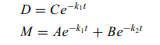# Develop a better model for ingestion of a tracer, which includes two separate systems: one system representing

Develop a better model for ingestion of a tracer, which includes two separate systems: one system representing the intestines (call it compartment 1) and another system representing the rest of the body (compartment 2).

a. Draw a block diagram showing the two systems with arrows connecting the inputs and outputs in appropriate ways.

b. Write a differential equation for the mass balance of tracer in each open system.

c. Show that the two equations are satisfied by functions of the form:where M is the amount of tracer in compartment 2 as a function of time, D is the amount of tracer in compartment 1 as a function of time, and A, B, and C are constant